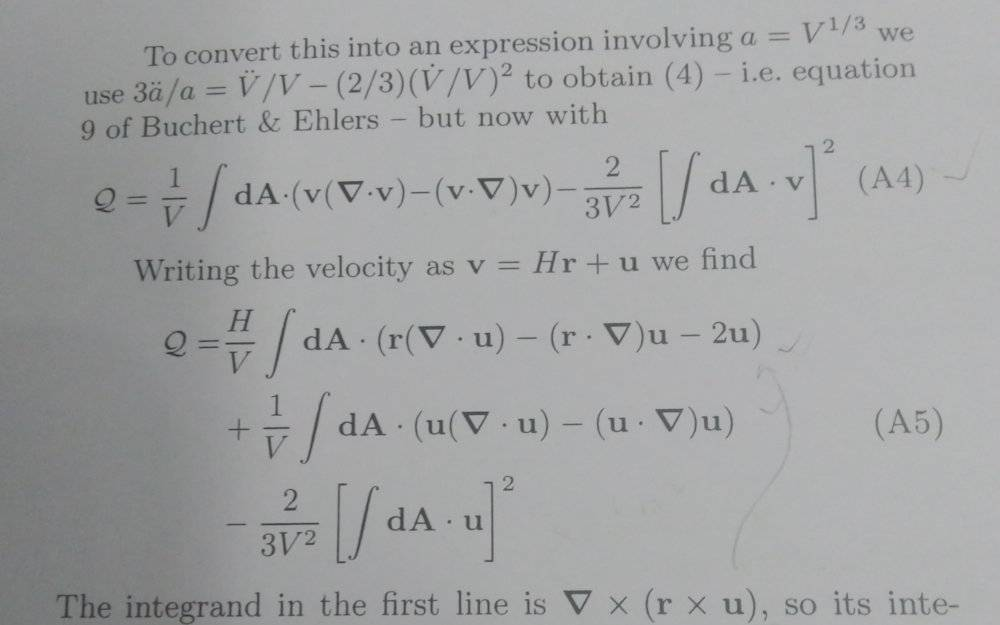# Is this surface integral correct?

Homework Statement:
Requesting for re check
Relevant Equations:
Requesting for re check
Problem Statement: Requesting for re check
Relevant Equations: Requesting for re checkIn this eq.A4 putting ##v=Hr+u## the first integrand in eq.A5 is coming as ##H(r(\nabla•u)-(r•\nabla)u+2u)\ne\nabla×(r×u)##
Am I right??
Can I request anyone to please recheck it.....
using this the author has put the term ##\int_s(da•\nabla×(r×u))=0##(as closed surface) how then it is coming??
##r## is the position vector and ##u ## is the peculiar velocity

Last edited:

TSny
Homework Helper
Gold Member
In this eq.A4 putting ##v=Hr+u## the first integrand in eq.A5 is coming as ##H(r(\nabla•u)-(r•\nabla)u+2u)\ne\nabla×(r×u)##
Am I right??
Shouldn't the last term on the left be ##-2u## instead of ##+2u##?

I'm not sure of the full meaning and context of the symbols. However, as a vector identity, I believe it is true that
$${\bf r} (\nabla \cdot {\bf u})-({\bf r} \cdot \nabla) {\bf u} -2 {\bf u}=\nabla×({\bf r} × {\bf u})$$

Last edited:
Shouldn't the last term on the left be ##-2u## instead of ##+2u##?

I'm not sure of the full meaning and context of the symbols. However, as a vector identity, I believe it is true that
$$r(\nabla•u)-(r•\nabla)u-2u=\nabla×(r×u)$$
Sir the last term is coming as ##2u## instead of ##-2u## e.g putting ##v=Hr+u## in eqA4 we get ##v(\nabla•v)=(Hr+u)(3H+\nabla•u)=3H^2r+Hr(\nabla•u)+3Hu+u(\nabla•u)##
Similarly ##-(v•\nabla)v=-[(Hr+u)•\nabla](Hr+u)=-(H^2r+H(r•\nabla)u+Hu+(u•\nabla)u)##
Similarly ##-\frac{2}{3V^2}[\int_s(da•v)]^2=-\frac{2}{3V^2}[\int_s(da•Hr)]^2-\frac{2}{3V^2}[\int(da•u)]^2=-6H^2-\frac{2}{3V^2}[\int(da•u)]^2##
Now adding these terms of the integrand we rest all term same except ##2Hu## instead of ##-2Hu## for which the author has simplified this to ##\nabla×(r×u)## and put it's surface integral to 0 (assuming closed surface) which is not coming true here....
That's what I want to clarify

TSny
Homework Helper
Gold Member
Similarly ##-\frac{2}{3V^2}[\int_s(da•v)]^2=-\frac{2}{3V^2}[\int_s(da•Hr)]^2-\frac{2}{3V^2}[\int(da•u)]^2##
There is a third term that should be included on the right side. Including this missing term should make everything OK.

There is a third term that should be included on the right side. Including this missing term should make everything OK.
Sorry I didn't get you

Sorry I didn't get you
Oh yes sir got it .....silly mistake
.....thanks